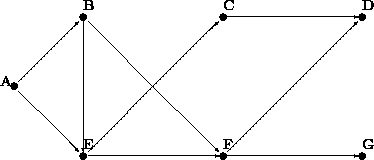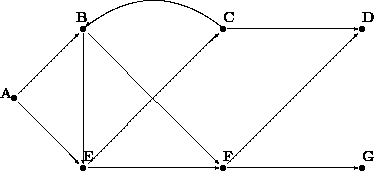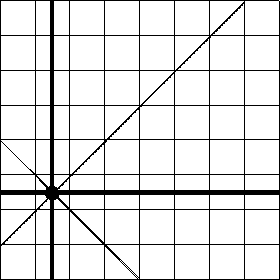# Section 28Algorithms that Backtrack

Solving problems does not always proceed on a direct route to the goal. Sometimes we make progress by pursuing one approach only to discover that we are stuck because we took a wrong turn. In those cases, we backtrack in our exploration and take a different turn at some branch, in the hope that it leads us to a solution. Algorithms can proceed like that. In the first subsection, we deal with an algorithm that can help us traverse a graph, which is of course the situation we just discussed. The second subsection is an extended exercise that uses backtracking in the context of chess.

## 28.1  Traversing Graphs

On occasion, we need to navigate through a maze of one-way streets. Or, we may wish to draw a graph of whom we consider a friend, whom they consider a friend, and so on. Or, we need to plan a route through a network of pipelines. Or, we ask the Internet to find some way to send a message from one place to another.

All these situations share a common element: a directed graph.

Specifically, there is always some collection of nodes and a collection of edges. The edges represent one-way connections between the nodes. Consider figure 76. The black bullets are the nodes; the arrows between them are the one-way connections. The sample graph consists of seven nodes and nine edges.

Now suppose we wish to plan routes in the graph of figure 76. For example, if we plan to go from C to D, the route is simple: it consists of the origination node C and the destination node D. In contrast, if we wish to travel from E to D, we have two choices:

1. We either travel from E to F and then to D.

2. Or, we travel from E to C and then to D.

For some nodes, however, it is impossible to connect them. In particular, it is impossible in our sample graph to move from C to G by following the arrows.Figure 76:  A directed graph

In the real world, graphs have more than just seven nodes and many more edges. Hence it is natural to develop functions that plan routes in graphs. Following the general design recipe, we start with a data analysis. Here is a compact representation of the graph in figure 76 using lists:

```(define Graph
'((A (B E))
(B (E F))
(C (D))
(D ())
(E (C F))
(F (D G))
(G ())))
```

The list contains one list per node. Each of these lists starts with the name of a node followed by the list of its neighbors. For example, the second list represents node B with its two outgoing edges to E and F.

Exercise 28.1.1.   Translate the above definition into proper list form using `list` and proper symbols.

The data definition for node is straightforward: A node is a symbol.

Formulate a data definition for graphs with arbitrarily many nodes and edges. The data definition must specify a class of data that contains `Graph`.Solution

Based on the data definitions for `node` and `graph`, we can now produce the first draft of a contract for `find-route`, the function that searches a route in a graph:

```;; `find-route : node node graph  ->  (listof node)`
;; to create a path from `origination` to `destination` in `G`
(define (find-route origination destination G) ...)
```

What this header leaves open is the exact shape of the result. It implies that the result is a list of nodes, but it does not say exactly which nodes the list contains. To understand this aspect, we must study some examples.

Consider the first problem mentioned above. Here is an expression that formulates the problem in Scheme:

```(find-route 'C 'D Graph)
```

A route from `'C` to `'D` consists of just two nodes: the origination and the destination node. Hence, we should expect the answer `(list 'C 'D)`. Of course, one might argue that since both the origination node and the destination node are known, the result should be `empty`. Here we choose the first alternative since it is more natural, but it requires only a minor change of the final function definition to produce the latter.

Now consider our second problem, going from `'E` to `'D`, which is more representative of the kinds of problems we might encounter. One natural idea is to inspect all of the neighbors of `'E` and to find a route from one of them to `'D`. In our sample graph, `'E` has two neighbors: `'C` and `'F`. Suppose for a moment that we didn't know the route yet. In that case, we could again inspect all of the neighbors of `'C` and find a route from those to our goal. Of course, `'C` has a single neighbor and it is `'D`. Putting together the results of all stages shows that the final result is `(list 'E 'C 'D)`.

Our final example poses a new problem. Suppose `find-route` is given the arguments `'C`, `'G`, and `Graph`. In this case, we know from inspecting figure 76 that there is no connecting route. To signal the lack of a route, `find-route` should produce a value that cannot be mistaken for a route. One good choice is `false`, a value that isn't a list and naturally denotes the failure of a function to compute a proper result.

This new agreement requires another change in our contract:

```;; `find-route : node node graph  ->  (listof node) or false`
;; to create a path from `origination` to `destination` in `G`
;; if there is no path, the function produces `false`
(define (find-route origination destination G) ...)
```

Our next step is to understand the four essential pieces of the function: the ``trivial problem'' condition, a matching solution, the generation of a new problem, and the combination step. The discussion of the three examples suggests answers. First, if the `origination` argument of `find-route` is equal to its `destination`, the problem is trivial; the matching answer is `(list destination)`. Second, if the arguments are different, we must inspect all neighbors of `origination` in `graph` and determine whether there is a route from one of those to `destination`.

Since a node can have an arbitrary number of neighbors, this task is too complex for a single primitive. We need an auxiliary function. The task of the auxiliary function is to consume a list of nodes and to determine for each one of them whether there is a route to the destination node in the given graph. Put differently, the function is a list-oriented version of `find-route`. Let us call this function `find-route/list`. Here is a translation of this informal description into a contract, header, and purpose statement:

```;; `find-route/list : (listof node) node graph  ->  (listof node) or false`
;; to create a path from some node on `lo-originations` to `destination`
;; if there is no path, the function produces `false`
(define (find-route/list lo-originations destination G) ...)
```

Now we can write a first draft of `find-route` as follows:

```(define (find-route origination destination G)
(cond
[(symbol=? origination destination) (list destination)]
[else ... (find-route/list (neighbors origination G) destination G) ...]))
```

The function `neighbors` generates a whole list of problems: the problems of finding routes from the neighbors of `origination` to `destination`. Its definition is a straightforward exercise in structural processing.

Exercise 28.1.2.   Develop the function `neighbors`. It consumes a node `n` and a graph `g` (see exercise 28.1.1) and produces the list of neighbors of `n` in `g`.Solution

Next we need to consider what `find-route/list` produces. If it finds a route from any of the neighbors, it produces a route from that neighbor to the final destination. But, if none of the neighbors is connected to the destination, the function produces `false`. Clearly, `find-route`'s answer depends on what `find-route/list` produces. Hence we should distinguish the answers with a cond-expression:

```(define (find-route origination destination G)
(cond
[(symbol=? origination destination) (list destination)]
[else (local ((define possible-route
(find-route/list (neighbors origination G)
destination G)))
(cond
[(boolean? route) ...]
[else ; `(cons? route)`
...]))]))
```

The two cases reflect the two kinds of answers we might receive: a boolean or a list. If `find-route/list` produces `false`, it failed to find a route from `origination`'s neighbors, and it is therefore impossible to reach `destination` at all. The answer in this case must therefore be `false`. In contrast, if `find-route/list` produces a list, the answer must be route from `origination` to `destination`. Since `possible-route` starts with one of `origination`'s neighbors, it suffices to add `origination` to the front of `possible-route`.

 ```;; `find-route : node node graph  ->  (listof node) or false` ;; to create a path from `origination` to `destination` in `G` ;; if there is no path, the function produces `false` (define (find-route origination destination G) (cond [(symbol=? origination destination) (list destination)] [else (local ((define possible-route (find-route/list (neighbors origination G) destination G))) (cond [(boolean? possible-route) false] [else (cons origination possible-route)]))])) ;; `find-route/list : (listof node) node graph  ->  (listof node) or false` ;; to create a path from some node on `lo-Os` to `D` ;; if there is no path, the function produces `false` (define (find-route/list lo-Os D G) (cond [(empty? lo-Os) false] [else (local ((define possible-route (find-route (first lo-Os) D G))) (cond [(boolean? possible-route) (find-route/list (rest lo-Os) D G)] [else possible-route]))])) ``` Figure 77:  Finding a route in a graph

Figure 77 contains the complete definition of `find-route`. It also contains a definition of `find-route/list`, which processes its first argument via structural recursion. For each node in the list, `find-route/list` uses `find-route` to check for a route. If `find-route` indeed produces a route, that route is the answer. Otherwise, if `find-route` fails and produces `false`, the function recurs. In other words, it backtracks its current choice of a starting position, `(first lo-Os)`, and instead tries the next one in the list. For that reason, `find-route` is often called a BACKTRACKING ALGORITHM.

Backtracking in the Structural World: Intermezzo 3 discusses backtracking in the structural world. A particularly good example is exercise 18.1.13, which concerns a backtracking function for family trees. The function first searches one branch of a family tree for a blue-eyed ancestor and, if this search produces `false`, it searches the other half of the tree. Since graphs generalize trees, comparing the two functions is an instructive exercise.

Last, but not least, we need to understand whether the function produces an answer in all situations. The second one, `find-route/list`, is structurally recursive and therefore always produces some value, assuming `find-route` always does. For `find-route` the answer is far from obvious. For example, when given the graph in figure 76 and two nodes in the graph, `find-route` always produces some answer. For other graphs, however, it does not always terminate.

Exercise 28.1.3.   Test `find-route`. Use it to find a route from A to G in the graph of figure 76. Ensure that it produces `false` when asked to find a route from C to G.Solution

Exercise 28.1.4.   Develop the function `test-on-all-nodes`, which consumes a graph `g` and tests `find-route` on all pairs of nodes in `g`. Test the function on `Graph`.SolutionFigure 78:  A directed graph with cycle

Consider the graph in figure 78. It differs radically from the graph in figure 76 in that it is possible to start a route in a node and to return to the same node. Specifically, it is possible to move from B to E to C and back to B. And indeed, if applied `find-route` to `'B`, `'D`, and a representation of the graph, it fails to stop. Here is the hand-evaluation:

```  (find-route 'B 'D Cyclic-graph)
= ... (find-route 'B 'D Cyclic-graph) ...
= ... (find-route/list (list 'E 'F) 'D Cyclic-graph) ...
= ... (find-route 'E 'D Cyclic-graph) ...
= ... (find-route/list (list 'C 'F) 'D Cyclic-graph) ...
= ... (find-route 'C 'D Cyclic-graph) ...
= ... (find-route/list (list 'B 'D) 'D Cyclic-graph) ...
= ... (find-route 'B 'D Cyclic-graph) ...
= ...
```

where `Cyclic-Graph` stands for a Scheme representation of the graph in figure 78. The hand-evaluation shows that after seven applications of `find-route` and `find-route/list` the computer must evaluate the exact same expression from which we started. Since the same input produces the same output and the same behavior for functions, we know that the function loops forever and does not produce a value.

In summary, if some given graph is cycle-free, `find-route` produces some output for any given inputs. After all, every route can only contain a finite number of nodes, and the number of routes is finite, too. The function therefore either exhaustively inspects all solutions starting from some given node or finds a route from the origination to the destination node. If, however, a graph contains a cycle, that is, a route from some node back to itself, `find-route` may not produce a result for some inputs. In the next part, we will study a programming technique that helps us finds routes even in the presence of cycles in a graph.

Exercise 28.1.5.   Test `find-route` on `'B`, `'C`, and the graph in figure 78. Use the ideas of section 17.8 to formulate the tests as boolean-valued expression.Solution

Exercise 28.1.6.   Organize the `find-route` program as a single function definition. Remove parameters from the locally defined functions.Solution

## 28.2  Extended Exercise: Checking (on) Queens

A famous problem in the game of chess concerns the placement of queens on a board. For our purposes, a chessboard is a ``square'' of, say, eight-by-eight or three-by-three tiles. The queen is a game piece that can move in a horizontal, vertical, or diagonal direction arbitrarily far. We say that a queen threatens a tile if it is on the tile or can move to it. Figure 79 shows an example. The solid disk represents a queen in the second column and sixth row. The solid lines radiating from the disk go through all those tiles that are threatened by the queen.Figure 79:  A chessboard with a single queen

The queen-placement problem is to place eight queens on a chessboard of eight-by-eight tiles such that the queens on the board don't threaten each other. In computing, we generalize the problem of course and ask whether we can place `n` queens on some board of arbitrary size `m` by `m`.

Even a cursory glance at the problem suggests that we need a data representation of boards and some basic functions on boards before we can even think of designing a program that solves the problem. Let's start with some basic data and function definitions.

Exercise 28.2.1.   Develop a data definition for chessboards.

Hint: Use lists. Represent tiles with `true` and `false`. A value of `true` should indicate that a position is available for the placement of a queen; `false` should indicate that a position is occupied by, or threatened by, a queen.Solution

Next we need a function for creating a board and another one for checking on a specific tile. Following the examples of lists, let's define `build-board` and `board-ref`.

Exercise 28.2.2.   Develop the following two functions on chessboards:

```;; `build-board : N (N N  ->  boolean)  ->  board`
;; to create a board of size `n` x `n`,
;; fill each position with indices `i` and `j` with `(f i j)`
(define (build-board n f) ...)

;; `board-ref : board N N  ->  boolean`
;; to access a position with indices `i`, `j` on a-board
(define (board-ref a-board i j) ...)
```

Test them rigorously! Use the ideas of section 17.8 to formulate the tests as boolean-valued expressions.Solution

In addition to these generic functions on a chessboard representation, we also need at least one function that captures the concept of a ``threat'' as mentioned in the problem statement.

Exercise 28.2.3.   Develop the function `threatened?`, which computes whether a queen can reach a position on the board from some given position. That is, the function consumes two positions, given as `posn` structures, and produces `true` if a queen on the first position can threaten the second position.

Hint: The exercise translate the chess problem of ``threatening queens'' into the mathematical problem of determining whether in some given grid, two positions are on the same vertical, horizontal, or diagonal line. Keep in mind that each position belongs to two diagonals and that the slope of a diagonal is either `+1` or `-1`.Solution

Once we have data definitions and functions for the ``language of chessboards,'' we can turn our attention to the main task: the algorithm for placing a number of queens on some given board.

Exercise 28.2.4.   Develop `placement`. The function consumes a natural number and a board and tries to place that many queens on the board. If the queens can be placed, the function produces an appropriate board. If not, it produces `false`.Solution